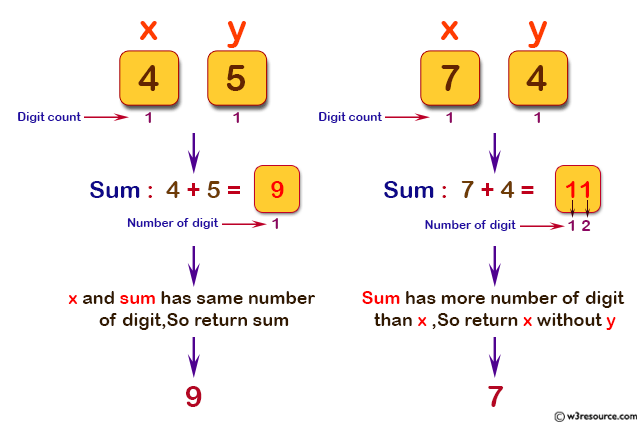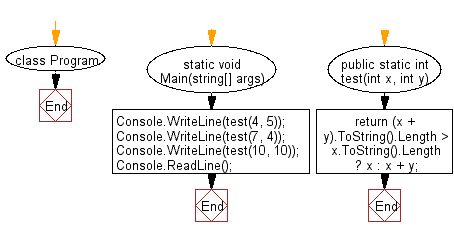﻿ C# - Sum of x and y must have the same number of digits as x# C# Sharp Basic Algorithm Exercises: Compute the sum of two given non-negative integers x and y as long as the sum has the same number of digits as x

## C# Sharp Basic Algorithm: Exercise-54 with Solution

Write a C# Sharp program to compute the sum of two given non-negative integers x and y as long as the sum has the same number of digits as x. If the sum has more digits than x then return x without y.

Pictorial Presentation:Sample Solution:-

C# Sharp Code:

``````using System;
using System.Linq;
namespace exercises
{
class Program
{
static void Main(string[] args)
{
Console.WriteLine(test(4, 5));
Console.WriteLine(test(7, 4));
Console.WriteLine(test(10, 10));
}

public static int test(int x, int y)
{
return (x + y).ToString().Length > x.ToString().Length ? x : x + y;
}
}
}
```
```

Sample Output:

```9
7
20```

Flowchart:C# Sharp Code Editor:

Improve this sample solution and post your code through Disqus

What is the difficulty level of this exercise?

Test your Programming skills with w3resource's quiz.

﻿